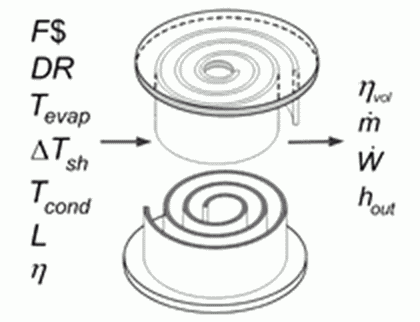Contents - index

Compressor4_CLCompressor4_CL is a model of a scroll compressor used for refrigeration and air conditioning applications.  The model is developed in terms of a leakage coefficient (L) to determine the volumetric efficiency and a overall efficiency used to determine the input power.  If one or both of these parameters are set to a negative values, default correlations are applied based on experimental data from:

Shrestha, S., Mahderekal, I, Sharma, V., and Abdelaziz, O.

Test Report #11

Compressor Calorimeter Test of  R-410A Alternatives R-32, DR-5,  and L-41a

Oak Ridge National Laboratory, April 5, 2013

and

Shrestha, S., Sharma, V., and Abdelaziz, O.

Test Report #33

Compressor Calorimeter Test of  R-410A Alternative: R-32/R-134a Mixture Using a Scroll Compressor

Oak Ridge National Laboratory, Feb. 18, 2014

Inputs:

F\$:  refrigerant

DR:  displacement rate  (m^3/s, ft^3/hr)

T_evap:  evaporating temperature (C, K, F, R)

DELTAT_sh: degrees of superheat at compressor inlet (DELTAK, DELTATF)

T_cond:  condensing temperature  (C, K, F, R)

L:  leakage fraction  (enter a value <0 to use default correlation)

eta:  combined isentropic efficiency of the compressor and electrical efficiency of the motor (enter value <0) for default correlation

Outputs:

eta_vol:  volumetric efficiency

m_dot:   mass flow rate of refrigerant (kg/s, lbm/hr)

W_dot:   compressor power (W)

h_out:  specific enthalpy at compressor exit

Example:

\$UnitSystem Eng F psia mass

F\$='R32'

DR=150.69 [ft^3/hr]

T_cond=120 [F]

T_evap=40 [F]

DELTAT_sh=40 [DELTAF]

L=-1  {use default correlation}

eta=-1 {use default correlation}

Call compressor4_cl(F\$, DR, T_evap, DELTAT_sh, T_cond,  L, eta: eta_vol, m_dot, W_dot, h_out)

{Solution:

eta_vol=0.8359 [-]

h_out=271.7 [Btu/lbm]

m_dot=174.7 [lbm/hr]

W_dot=1982 [W]

}